# Finding the area of a right triangle or its corresponding rectangle Online Quiz

Following quiz provides Multiple Choice Questions (MCQs) related to Finding the area of a right triangle or its corresponding rectangle. You will have to read all the given answers and click over the correct answer. If you are not sure about the answer then you can check the answer using Show Answer button. You can use Next Quiz button to check new set of questions in the quiz.Q 1 - Find the area of following right triangle and its corresponding rectangle.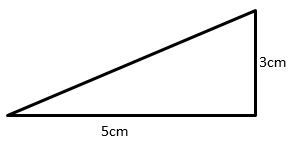### Explanation

Step 1:

Area of Right Triangle = $\frac{1}{2}$ × b × h; b = base = 5; h = height = 3

Step 2:

Area of Right Triangle = $\frac{1}{2}$ × 5 × 3 = 7.5 square cm

Area of corresponding rectangle = b × h = 5 × 3 = 15 square cm

Q 2 - Find the area of following right triangle and its corresponding rectangle.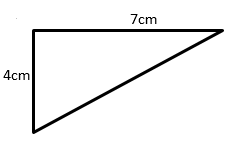### Explanation

Step 1:

Area of Right Triangle = $\frac{1}{2}$ × b × h; b = base = 4; h = height = 7

Step 2:

Area of Right Triangle = $\frac{1}{2}$ × 4 × 7 = 14 square cm

Area of corresponding rectangle = 4 × 7 = 28 square cm

Q 3 - Find the area of following right triangle and its corresponding rectangle.### Explanation

Step 1:

Area of Right Triangle = $\frac{1}{2}$ × b × h; b = base = 6; h = height = 3

Step 2:

Area of Right Triangle = $\frac{1}{2}$ × 6 × 3 = 9 square cm

Area of corresponding rectangle = b × h = 6 × 3 = 18 square cm

Q 4 - Find the area of following right triangle and its corresponding rectangle.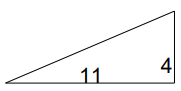### Explanation

Step 1:

Area of Right Triangle = $\frac{1}{2}$ × b × h; b = base = 11; h = height = 4

Step 2:

Area of Right Triangle = $\frac{1}{2}$ × 11 × 4 = 22 square units

Area of corresponding rectangle = b × h = 11 × 4 = 44 square units

Q 5 - Find the area of following right triangle and its corresponding rectangle.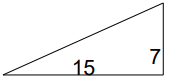### Explanation

Step 1:

Area of Right Triangle = $\frac{1}{2}$ × b × h; b = base = 15; h = height = 7

Step 2:

Area of Right Triangle = $\frac{1}{2}$ × 15 × 7 = 52.5 square units

Area of corresponding rectangle = b × h = 15 × 7 = 105 square units

Q 6 - Find the area of following right triangle and its corresponding rectangle.### Explanation

Step 1:

Area of Right Triangle = $\frac{1}{2}$ × b × h; b = base = 12; h = height = 5

Step 2:

Area of Right Triangle = $\frac{1}{2}$ × 12 × 5 = 30 square units

Area of corresponding rectangle = b × h = 12 × 5 = 60 square units

Q 7 - Find the area of following right triangle and its corresponding rectangle.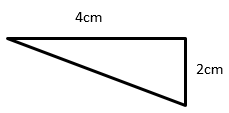### Explanation

Step 1:

Area of Right Triangle = $\frac{1}{2}$ × b × h; b = base = 4; h = height = 2

Step 2:

Area of Right Triangle = $\frac{1}{2}$ × 4 × 2 = 4 square cm

Area of corresponding rectangle = b × h = 4 × 2 = 8 square cm

Q 8 - Find the area of following right triangle and its corresponding rectangle.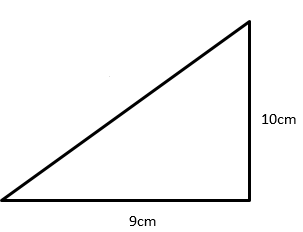### Explanation

Step 1:

Area of Right Triangle = $\frac{1}{2}$ × b × h; b = base = 10; h = height = 9

Step 2:

Area of Right Triangle = $\frac{1}{2}$ × 10 × 9 = 45 square cm

Area of corresponding rectangle = b × h = 10 × 9 = 90 square cm

Q 9 - Find the area of following right triangle and its corresponding rectangle.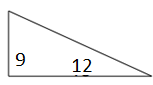### Explanation

Step 1:

Area of Right Triangle = $\frac{1}{2}$ × b × h; b = base = 12; h = height = 9

Step 2:

Area of Right Triangle = $\frac{1}{2}$ × 12 × 9 = 54 square units

Area of corresponding rectangle = b × h = 12 × 9 = 108 square units

Q 10 - Find the area of following right triangle and its corresponding rectangle.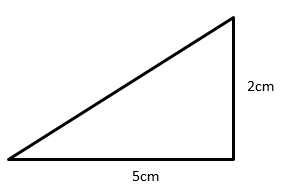### Explanation

Step 1:

Area of Right Triangle = $\frac{1}{2}$ × b × h; b = base = 5; h = height = 2

Step 2:

Area of Right Triangle = $\frac{1}{2}$ × 5 × 2 = 5 square cm

Area of corresponding rectangle = b × h = 5 × 2 = 10 square cm

finding_area_of_right_triangle_or_its_corresponding_rectangle.htm